Important Surface Area Questions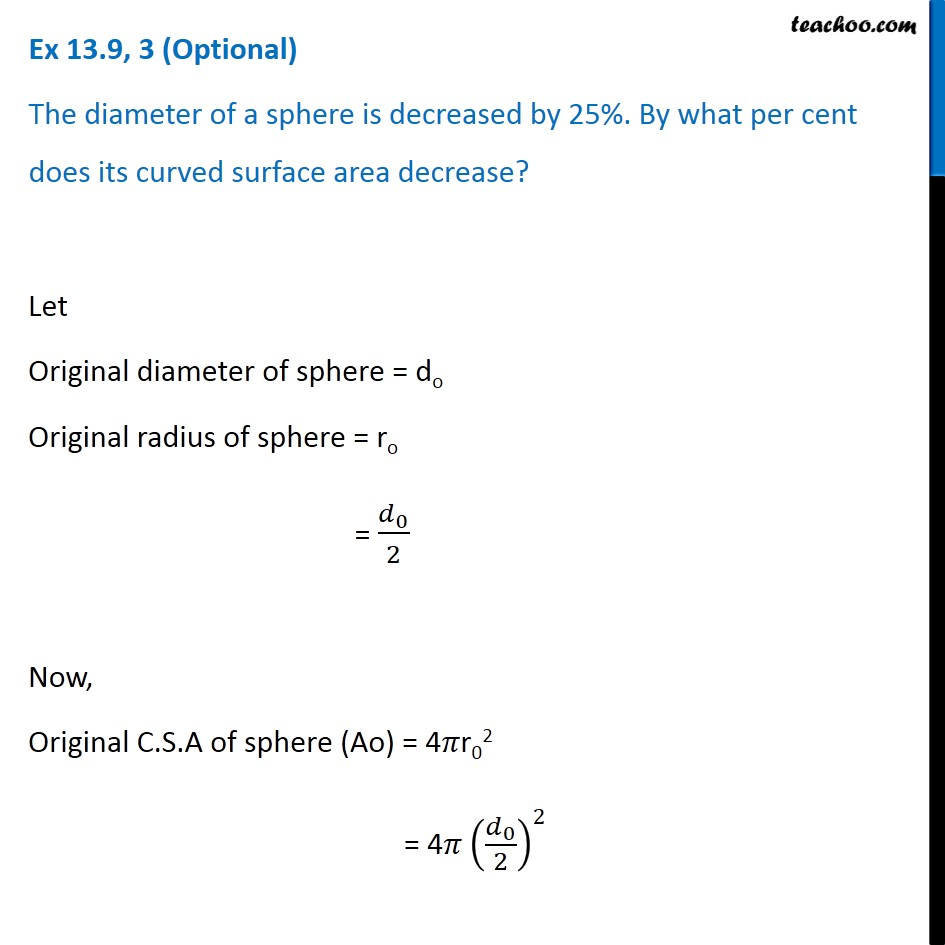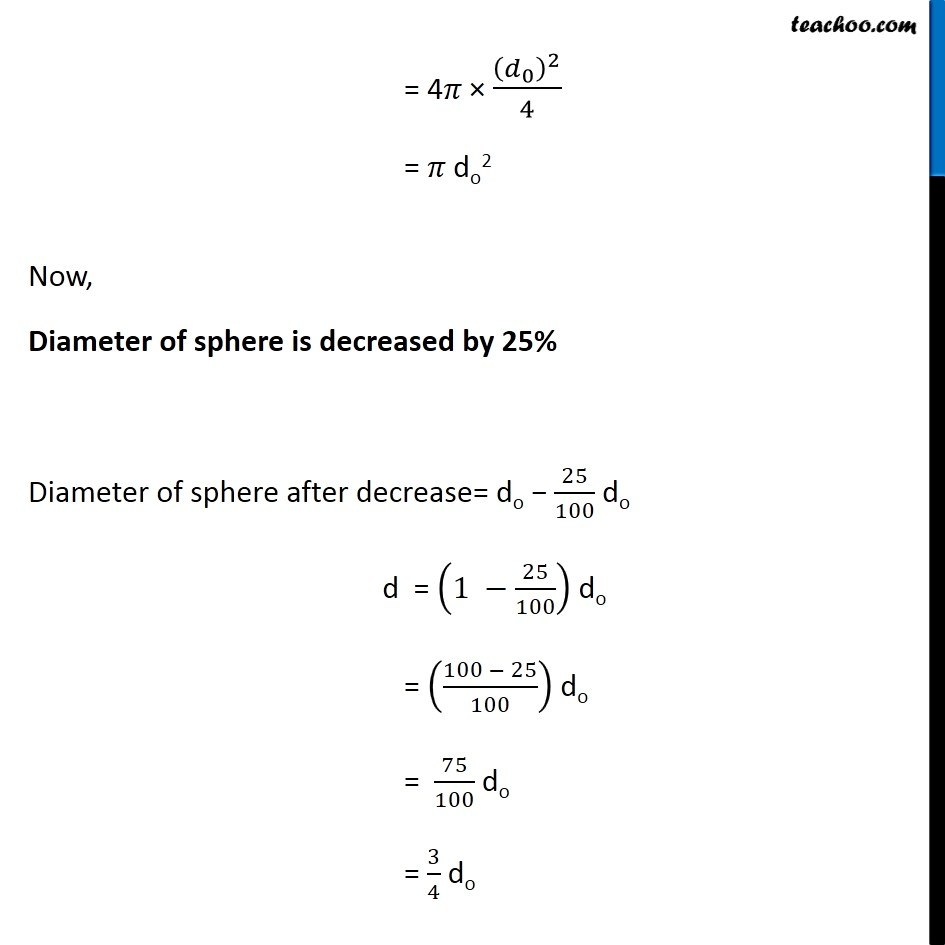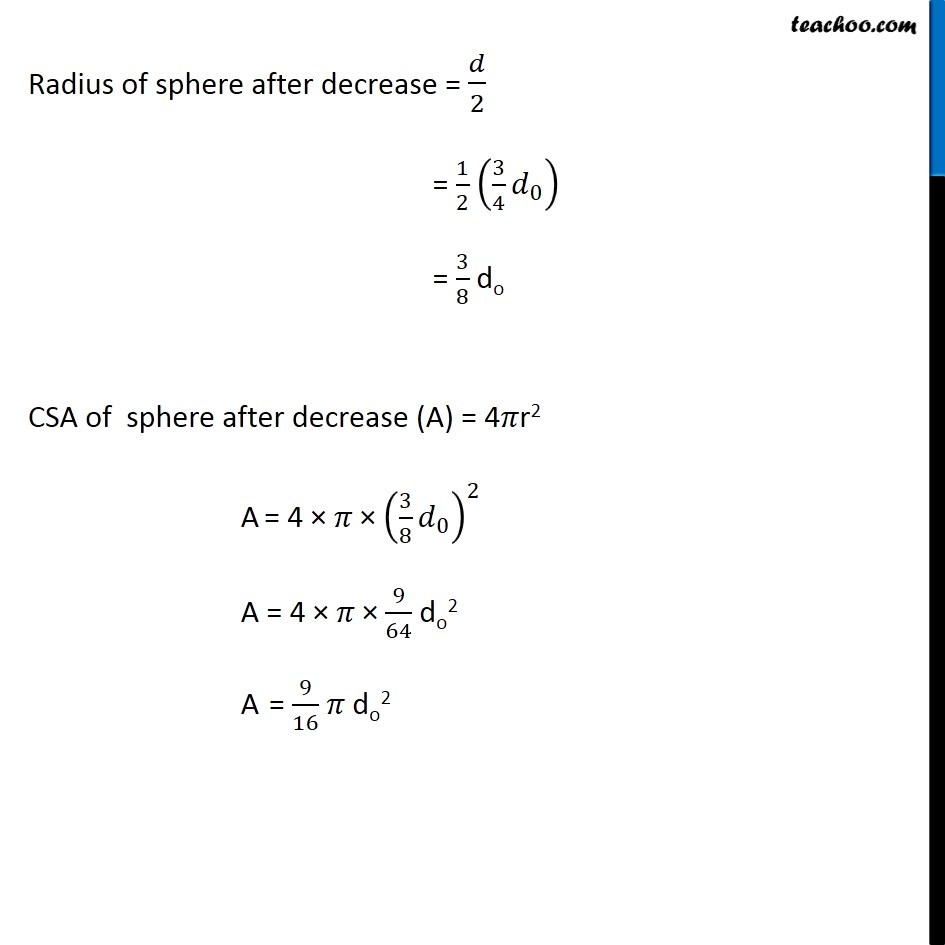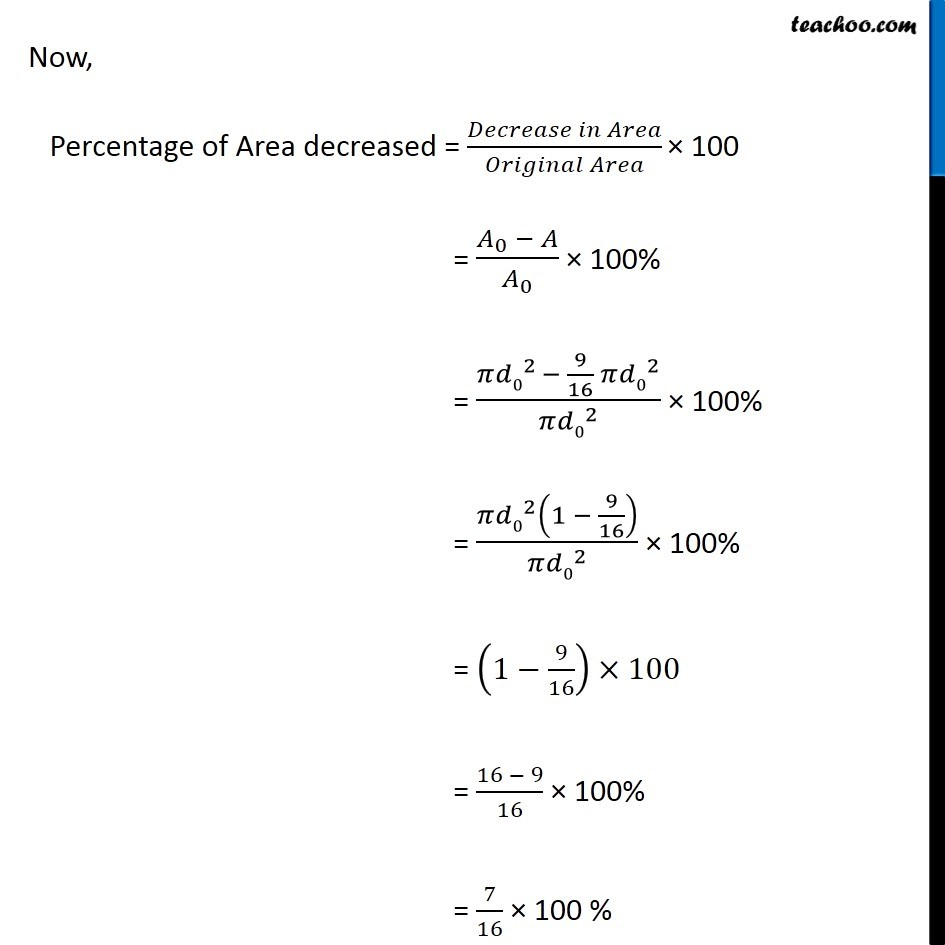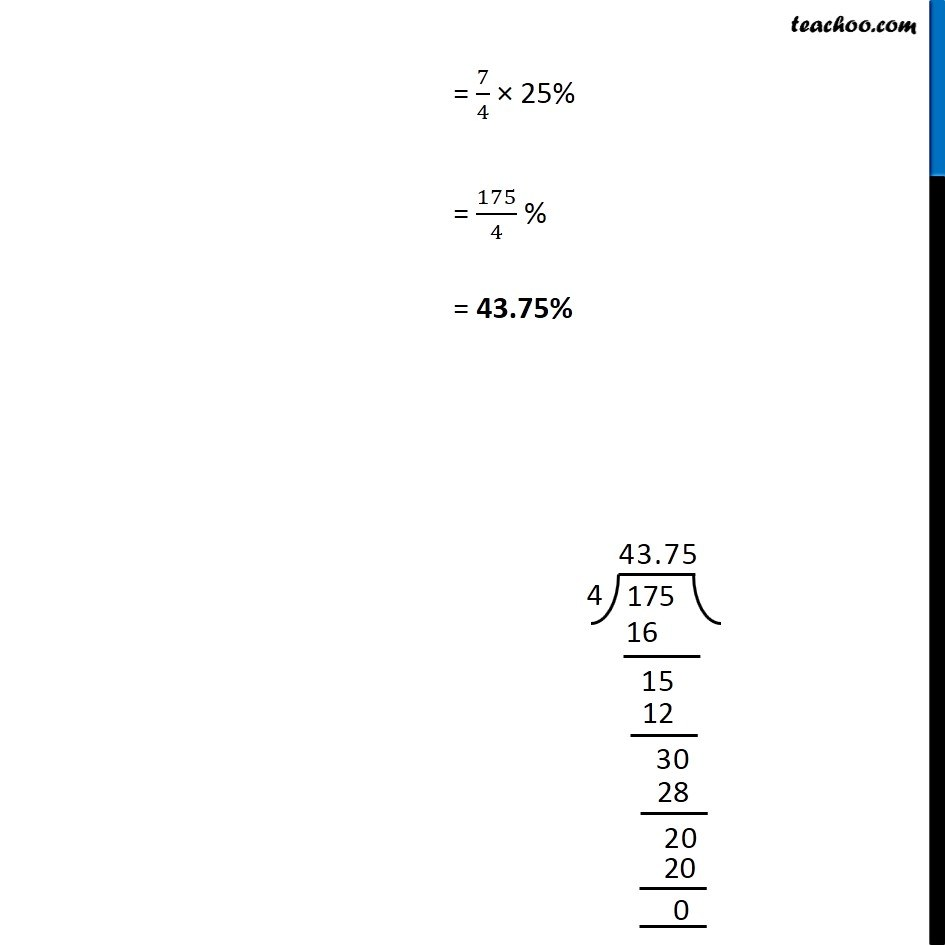Learn in your speed, with individual attention - Teachoo Maths 1-on-1 Class

### Transcript

Question 3 The diameter of a sphere is decreased by 25%. By what per cent does its curved surface area decrease? Let Original diameter of sphere = do Original radius of sphere = ro = 𝑑_0/2 Now, Original C.S.A of sphere (Ao) = 4𝜋r02 = 4𝜋 (𝑑_0/2)^2 = 4𝜋 × (𝑑_0 )^2/4 = 𝜋 do2 Now, Diameter of sphere is decreased by 25% Diameter of sphere after decrease= do − 25/100 do d = (1 −25/100) do = ((100 − 25)/100) do = 75/100 do = 3/4 do Radius of sphere after decrease = 𝑑/2 = 1/2 (3/4 𝑑_0 ) = 3/8 do CSA of sphere after decrease (A) = 4𝜋r2 A = 4 × 𝜋 × (3/8 𝑑_0 )^2 A = 4 × 𝜋 × 9/64 do2 A = 9/16 𝜋 do2 Now, Percentage of Area decreased = (𝐷𝑒𝑐𝑟𝑒𝑎𝑠𝑒 𝑖𝑛 𝐴𝑟𝑒𝑎)/(𝑂𝑟𝑖𝑔𝑖𝑛𝑎𝑙 𝐴𝑟𝑒𝑎) × 100 = (𝐴_0 − 𝐴)/𝐴_0 × 100% = (𝜋〖𝑑0〗^2 − 9/16 𝜋〖𝑑0〗^2)/(𝜋〖𝑑0〗^2 ) × 100% = (𝜋〖𝑑0〗^2 (1 − 9/16))/(𝜋〖𝑑0〗^2 ) × 100% = (1−9/16)×100 = (16 − 9)/16 × 100% = 7/16 × 100 % = 7/4 × 25% = 175/4 % = 43.75% 43.75 4 175 16 15 12 30 28 20 20 0